## Garden Railway Construction

I got permission from the boss to build a garden railway in our backyard largely because I am going to put it in the previously uncivilized portion of the yard. So, step one is to civilize the wild parts.

A couple of years ago, I began an ivy eradication project. There must have been 1,000 square feet of ivy, which made that area useless for anything. So, repeated applications of Bayer Advanced Brush Killer has wiped out most of the ivy and some other brush from our yard. (I’m on my 3rd container of Brush Killer.)

Now, I could tackle the wild iris, pyracantha, hawthorn and blackberry canes. Here’s a couple of before photos: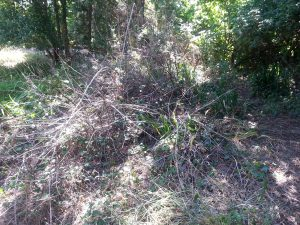You can see the wild iris on the left, and the massive tangle of brush that I had to contend with. Note also the remnants of (very sickly) ivy. After about a month, here is my progress: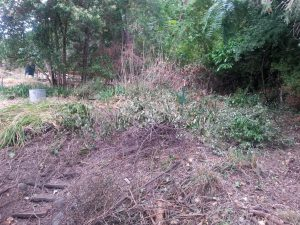I still have quite a bit of brush, but it is no longer attached to the ground. And I’ve beaten the iris back to less than half what it was. In a few weeks, the sticks and iris will all be gone.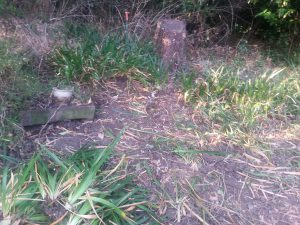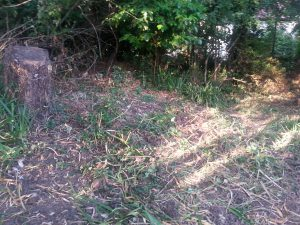Note the progress on the wild iris (before and after, above), and the elimination of some holly sticks that were left after the brush killer. As a bonus, friends are taking the dug-up iris away to plant on their property.Update: Here is the garden area, almost bare ground where there was all that ivy and brush. This represents two to three months of hacking and raking.

## One more Javascript function

This function will format a value expressed as feet (with decimals) into feet and inches.

```// Convert feet (decimal) into feet and inches
function toString(value)
{
var abs = Math.abs(value);
// Round to nearest 0.1 inch
var rounded = Math.round(120 * abs) / 120;

var feet = Math.floor(rounded);
var inches = 12*(rounded - feet);

return (value < 0 ? "-" : "")
+ (feet != 0 ? feet + "'" : "")
+ (inches.toFixed(1) + '"');
}
```

## Perfect Hard-boiled EggsPlace eggs into salted water that covers them by at least 1/2 inch.Bring to a good boil, remove from heat and cover them. Let sit for 15 minutes. One of the eggs developed a tiny crack, and lost some white. No problemo!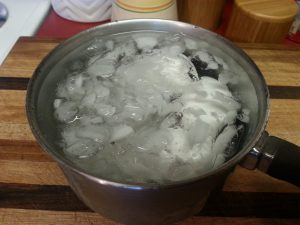After they have cooked, drain the hot water and use ice and water to chill the eggs thoroughly.To peel them, crack the shell slightly all over the egg. Put them one at a time into a container with about 1 inch of water. The container should be large enough that the egg has plenty of room to move.Put the lid on and shake the container vigorously. The water will help to prevent damage to the egg and float the shell away from the egg.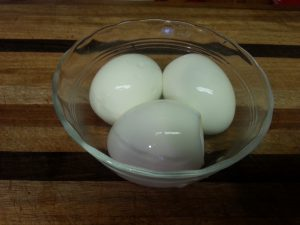Here they are, after about 1 minute of work. And note that the yolks are perfectly done.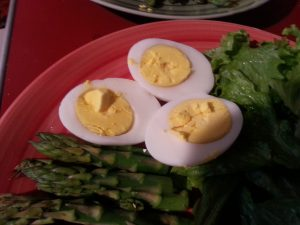## Javascript functions for numeric input and output

As a part of a calculator for model railroad stub switches, I developed a couple of javascript routines for input and output that allow more flexibility than the standard implementation.

This function converts to text for display from a numeric value, with a variable number of decimals, removing extra trailing zeroes. It also converts the (default inch) measure to millimeters based on the units global variable.

```// Smarter formatting function value, decimals
function toVariable(v, d)
{
if (units == "mm")
v = v * 25.4;
var r = v.toFixed(d).replace(/0+\$/g, '');
if (r.substring(r.length - 1) == '.')
{
r = r.substring(0, r.length - 1);
}
return r;
}
```

This function accepts text from an input box in various formats and units of measurement. The return value is always converted into inches.
Value formats:

• Decimal: a value such as 1.5
• Mixed fraction: a value such as 1 1/2
• Fraction: a value such as 3/8
• Decimal: a value such as 0.375

Measure specifiers:

• in: inches
• ft: feet
• mm: millimeter
• cm: centimeter
```// Parse a measurement in mm, cm, in or ft
// Always returns the value converted to inches
function parseMeasure(text)
{
// Mixed ex: 1 1/2 in
var re = /(\d+)\s+(\d+)\/(\d+)\s*(mm|cm|in|ft)?\.?/;
var matches = text.match(re);

var value;
var num, den;
var measure;

if (matches && matches.length > 0)
{
value = matches;
num = matches;
den = matches;
measure = (matches.length > 4 ? matches : "in");
}
else
{
// Fraction ex: 1/2 in
re = /(\d+)\/(\d+)\s*(mm|cm|in|ft)?\.?/;
matches = text.match(re);

if (matches && matches.length > 0)
{
value = "0";
num = matches;
den = matches;
measure = (matches.length > 3 ? matches : "in");
}
else
{
// Decimal ex: 1.5 in
re = /(\d+\.?\d*)\s*(mm|cm|in|ft)?\.?/;
matches = text.match(re);

var value = (matches.length > 1 ? matches : "0");
var measure = (matches.length > 2 ? matches : "in");
}
}

// Add the fraction part, if any
var x = parseFloat(value);
if (num && den)
{
x = x + parseFloat(num) / parseFloat(den);
}

// Convert to inches
var r = x;
switch (measure)
{
case "mm":
r = x / 25.4;
break;
case "cm":
r = x / 2.54;
break;
case "in":
r = x;
break;
case "ft":
r = x * 12;
break;
}

return r;
}
```